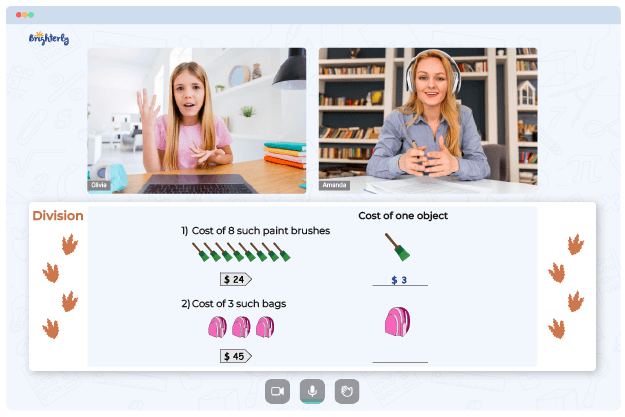# Multiplying And Dividing Fractions Worksheets

Understanding mathematical concepts may be difficult for kids and depends on a tutor’s approach. The solution to successful math learning comes from creative resources like worksheets and games. For kids learning division and multiplication of fractions, the use of multiplying and dividing fractions worksheets is a suitable and seamless model for learning.

## Free Multiplication and Division of Fractions Worksheets from Brighterly

Brighterly’s dividing and multiplying worksheets present a fantastic way to help kids understand the concepts of fractions, multiplication, and division. Multiplication and division of fractions can be difficult for kids; hence, having material they can study and revisit at their convenience is essential.

The worksheets are packed with real-life problems and images capable of seizing kids’ attention. Since the target population behind the creation of the worksheets are kids, the tools are designed to address their needs. Furthermore, Brighterly’s worksheets are designed by top professionals with years of experience in handling and teaching kids.

Math for Kids

Is Your Child Struggling With Math?
1:1 Online Math TutoringYou can take advantage of tons of dividing and multiplying fraction worksheets on Brighterly’s website that can make learning seamless for your kids. You can get numerous printable multiplying and dividing fractions worksheets in pdf to ensure your kids can learn anytime.

## Why You Need a Multiply and Divide Fractions Worksheet

The benefits of using the Brighterly’s divide and multiply fraction worksheets are numerous for students, educators, and parents. Below are some of the pros of using this fantastic math learning tool.

### Worksheets help kids learn the meaning of concepts

The worksheets help students understand the meaning and importance of fractions, multiplication, and division, which boosts students’ desire to learn and make understanding of complicated topics easier.### Multiplying And Dividing Fractions Worksheets PDF

Multiplying And Dividing Fractions Worksheets### Multiplying And Dividing Fractions Worksheets PDF

Multiplying And Dividing Fractions Worksheet### Multiplying And Dividing Fractions Worksheets PDF

Multiplication And Division Of Fractions Worksheets### Multiplying And Dividing Fractions Worksheets PDF

Multiply And Divide Fractions Worksheet

### They make learning fun

Using multiplying and dividing fractions worksheets, kids are able to learn while having fun. The sheets contain manipulatives and exciting graphics to capture your child’s attention.

Helping your kid with online math lessons for 4th grade. Expert tutors inspiring kids to love math so they can excel at It.

### The tools reduce the workload of educators

Multiplying and dividing mixed fractions worksheets with answers can help your kids learn independently, even without supervision. So, you don’t need to spend the whole session teaching the concepts.

### Fractions Worksheets

Troubles with Division?• Does your child struggle to grasp the concept of division?
• Try learning with an online tutor.

Is your child having difficulties with understanding division? An online tutor could provide the necessary help.